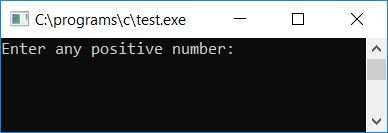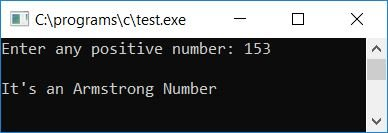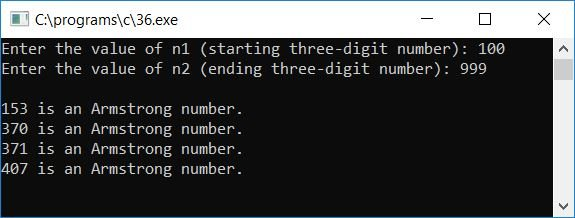# C Program to Check the Armstrong Number

In this article, you will learn and get code for checking whether the given number by the user (at run-time) is an Armstrong number or not. But before going through the program, Let's first understand what an Armstrong number is.

### How to Find an Armstrong Number

For a number to be an Armstrong number, the sum of the cubes of all of its digits must be equal to the number itself. For example, 153 is an Armstrong number. Because

• 153 = (1*1*1) + (5*5*5) + (3*3*3)

As you can clearly see, the sum of the cubes of all of its digits (1, 5, and 3) is equal to the number itself. So it is an Armstrong number. But the number 26  is not an Armstrong number. Because

• 26 != (2*2*2) + (6*6*6)

Now let's move on and implement it in a C program.

## Check if the given number is an Armstrong number in C

The question is, "Write a program in C to check whether the given number is an Armstrong number or not." The answer to this question is:

```#include<stdio.h>
#include<conio.h>
int main()
{
int n, nu, num=0, rem;
printf("Enter any positive number: ");
scanf("%d", &n);
nu=n;
while(nu!=0)
{
rem = nu%10;
num = num + (rem*rem*rem);
nu = nu/10;
}
if(num==n)
printf("\nIt's an Armstrong Number");
else
printf("\nIt's not an Armstrong Number");
getch();
return 0;
}```

The above program was written in the Code::Blocks IDE; therefore, after a successful build and run, here is the output you will get on your output screen:Now supply any positive number, say 153, and press the ENTER key to see the output that will say whether it is an Armstrong number or not, as shown in the following snapshot:#### Program Explained

• Get any positive number as input from the user, say 153.
• Now initialize the number to another variable, say nu, for the operation.
• Include a while loop to work with each and every digit of the given number, say 153.
• We have to find the remainder of the number one by one. The first remainder we will get is 3.
• Initialize 3 to the rem variable, calculate the cube of the rem, and initialize it to the num variable after summing it with the variable itself.
• At the start of the program, we set the num variable to 0.
• Divide the number nu by ten.
• Also, ensure that the value inside nu is not equal to 0.
• If the condition is met, the while loop is re-entered and do the same operation until nu holds the value 0.
• Therefore, at the first run of the while loop, rem holds 3, num holds 0 + 3*3*3 or 27, and nu holds 15. And at the second run of the while loop, rem holds 5, num holds 27 + 5*5*5 or 152, and nu holds 1. In the third run, rem is 1, num is 152 + 1*11*1 or 153, and nu is 0.
• At last, check whether the value of num is equal to the given number (the value present inside the variable n) or not.
• If it is equal, then the number is an Armstrong number; otherwise, the number is not an Armstrong number.

Here is another program in C that prints all Armstrong numbers between any two given three-digit numbers. To generate or print Armstrong numbers without caring about digits, refer to Generate Armstrong Numbers.

```#include<stdio.h>
#include<conio.h>
int main()
{
int n1, n2, i, temp, rem, sum, prod;
printf("Enter the value of n1 (starting three-digit number): ");
scanf("%d", &n1);
printf("Enter the value of n2 (ending three-digit number): ");
scanf("%d", &n2);
printf("\n");
for(i=n1; i<=n2; i++)
{
sum = 0;
temp = i;
while(temp>0)
{
rem = temp%10;
sum = sum + (rem*rem*rem);
temp = temp/10;
}
if(sum == i)
printf("%d is an Armstrong number.\n", i);
}
getch();
return 0;
}```

Here is the sample run:Now provide any three-digit number as the starting point, say 100, and another three-digit number as the ending point, say 999, to print all the Armstrong numbers present in between these two numbers. Here is the second snapshot of the sample run:The logic in this program is nearly identical to that in the previous one, except that we have used a for loop to begin with n1 (the starting number) and end with n2 (the ending number).In between these two numbers, we have used the same concept inside the for loop, namely, if the number is found to be an Armstrong number, then print it out, otherwise get to the next one to check for Armstrong.

Before checking, initialize 0 to sum and i to the temp variable for the operation. Here we have used the sum variable in place of the num variable. You can choose something different; it is up to you.

#### The same program in different languages

C Quiz

« Previous Program Next Program »